# Lid Driven Cavity Flow¶

## Configuration¶

### 1. We want to have a lid driven cavity flow with (according to the QPF) :¶

Small Reynolds Number

Re = 0.000061

```dom = DomainRectFluid(nu_=1.0, dimx=0.82, dimy=0.61, delta_x=0.02)
vel = 0.0001

cfd_lid_driven(10, 0.30)
```

### 2. Works also with another configuration to get another Reynolds number :¶

High Reynolds Number

Re = 300

```size = 1.0
dom = DomainRectFluid(dimx=size, dimy=size, delta_x=size / 60, rho=1.0, nu_=0.01)

vel = 3.0
```

Because we are at high Reynolds number :

• we need to have an important t_end

• Moreover to avoid the following error:

```  RuntimeError: too many sub iterations 10101
```

one can put more saving moments to have weaker iterations.

## Reference case¶

### the script¶

```	"""Example on how to solve a Lid driven cavity problem with the navier stokes solver"""

from barbatruc.fluid_domain import DomainRectFluid
from barbatruc.fd_ns_2d import NS_fd_2D_explicit

# from barbatruc.lattice import Lattice

__all__ = ["cfd_lid_driven"]

# pylint: disable=duplicate-code
def cfd_lid_driven(nsave, tchar):
"""Startup computation
solve a lid_driven_cavity  problem
"""
size = 1.0
dom = DomainRectFluid(dimx=size, dimy=size, delta_x=size / 60, rho=1.0, nu_=0.01)

vel = 3.0
t_end = tchar * size / vel
dom.switch_bc_xmin_wall_noslip()
dom.switch_bc_xmax_wall_noslip()
dom.switch_bc_ymax_moving_wall(vel_u=vel)
dom.switch_bc_ymin_wall_noslip()

time = 0.0
time_step = t_end / nsave

# solver = Lattice(dom, max_vel=2*vel)
solver = NS_fd_2D_explicit(
dom, obs_ib_factor=0.01, press_maxsteps=200, press_tol=5.0e-3, max_vel=4.0
)
for i in range(nsave):
time += time_step
solver.iteration(time, time_step)
print("\n\n===============================")
print(f"\n  Iteration {i+1}/{nsave}, Time :, {time}s")
print(f"  Reynolds : {dom.reynolds(size)}")
print(dom.fields_stats())
dom.dump_paraview(time=time)
dom.dump_global(time=time)
dom.show_fields()
dom.show_flow()
dom.show_profile_y(xtgt=0.41)
print("Normal end of execution.")

if __name__ == "__main__":
cfd_lid_driven(10, 10)
```

## For Re = 0.000061¶

### The flow output :¶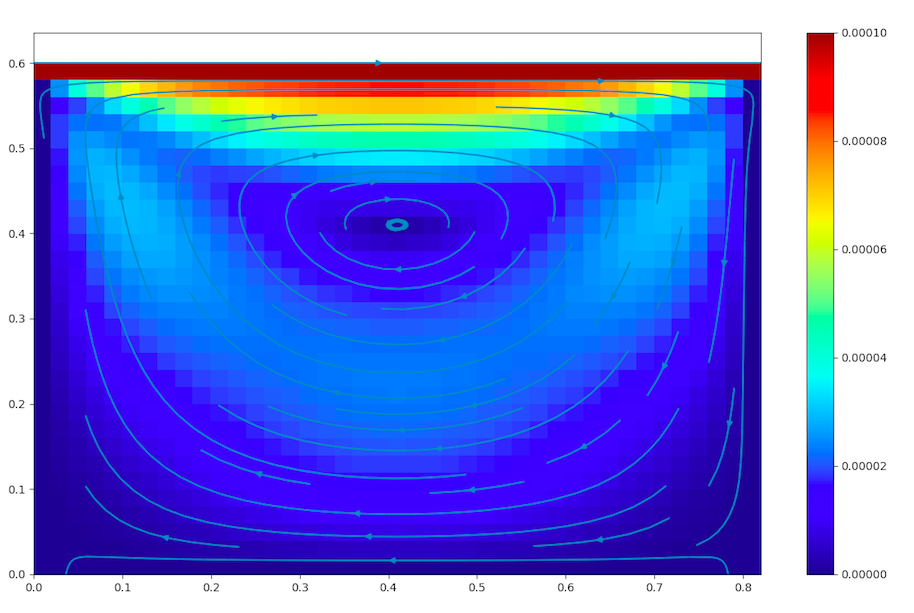cavityflow

### The fields :¶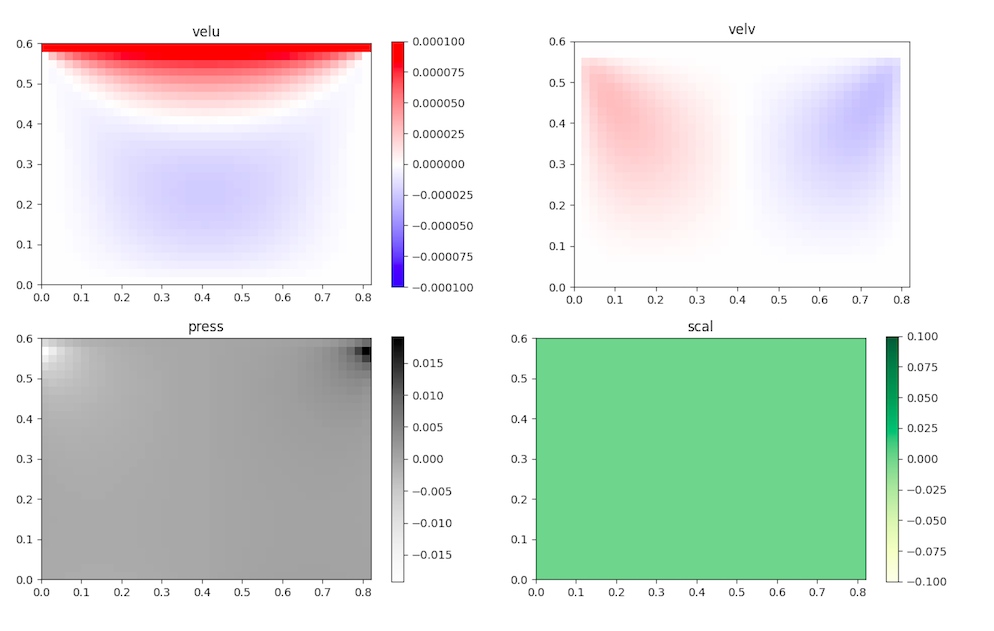cavityfields

### The velocity profile :¶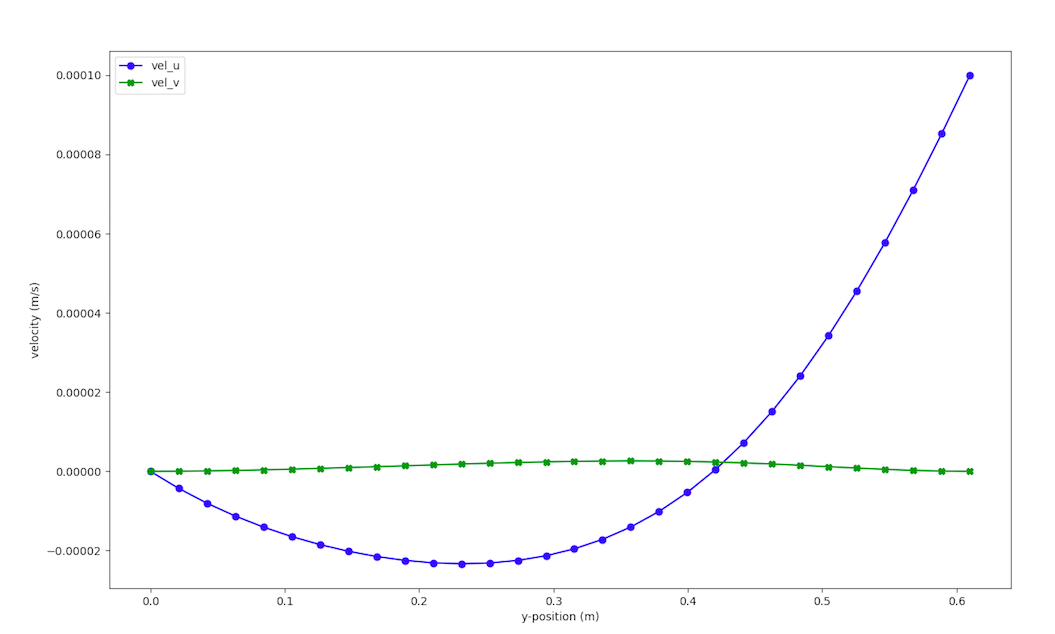cavityprofile

## For Re = 300¶

### The flow output :¶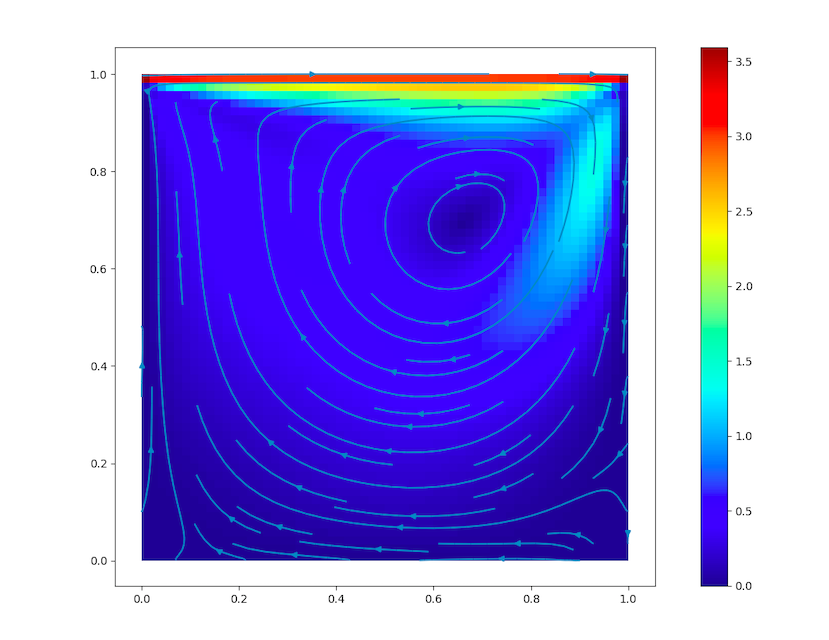cavity300flow

### The fields :¶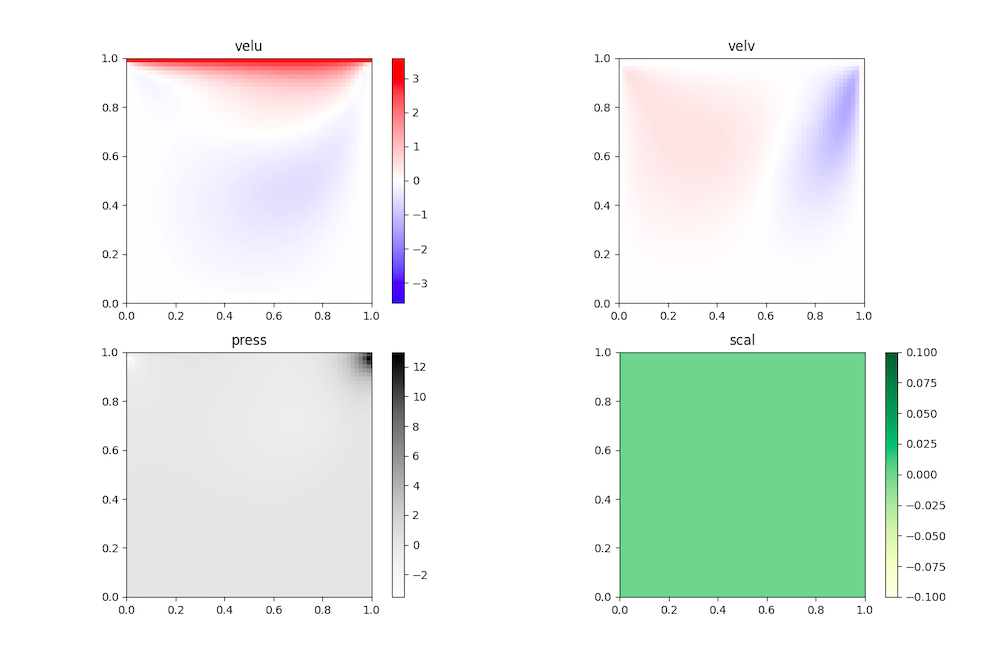cavityfields300

### The velocity profile :¶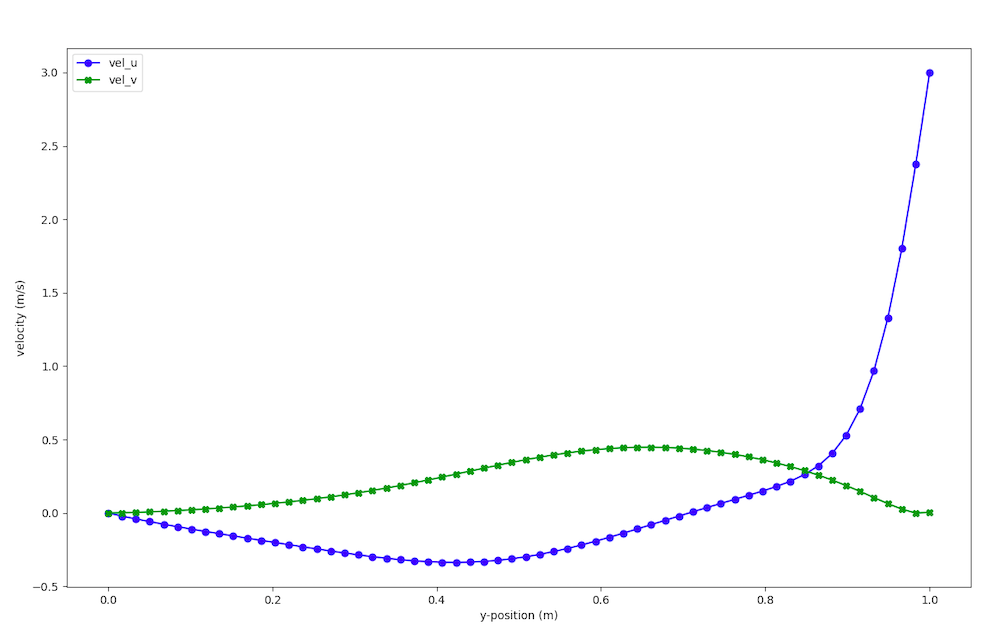cavityeprofile300

### Solver parameters:¶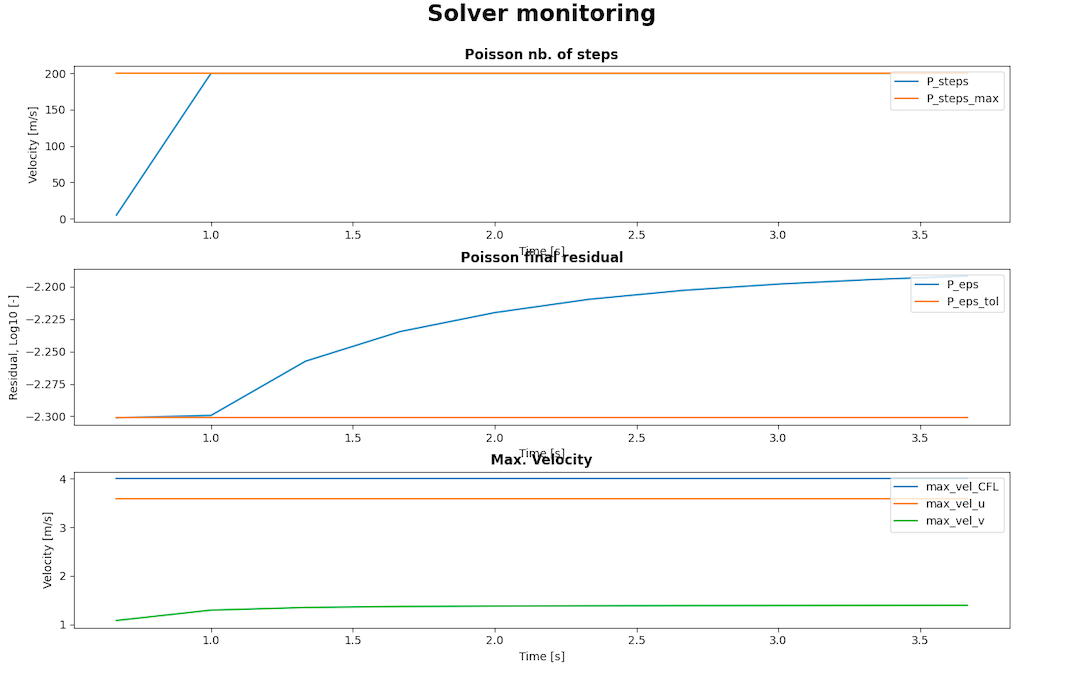cavitysolver

Before modifications:

```  solver = NS_fd_2D_explicit(dom, obs_ib_factor=0.9, max_vel=2*vel)
```

To make the residual better we used a small delta_x and we manipulated the different parameters of the solver until having satisfactory results:

```	  solver = NS_fd_2D_explicit(
dom, obs_ib_factor=0.01, press_maxsteps=200, press_tol=5.0e-3, max_vel=4.0)
```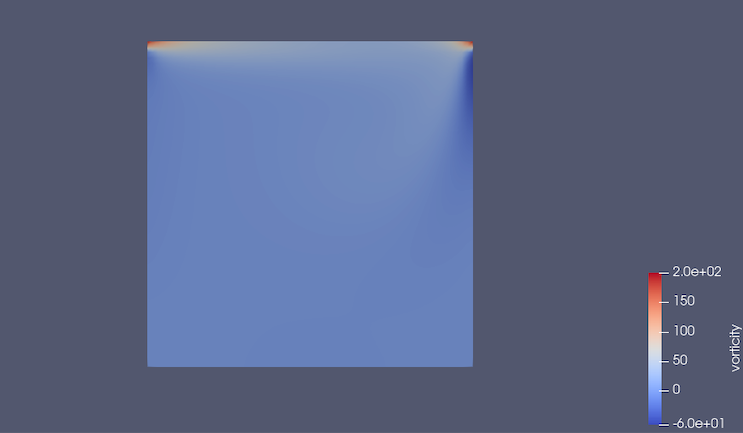cavityVORTICITY

There is a high vorticity at the top corners, because they are singularities and the solver has difficulties to converge.

## Comparison with the documentation for Re = 100¶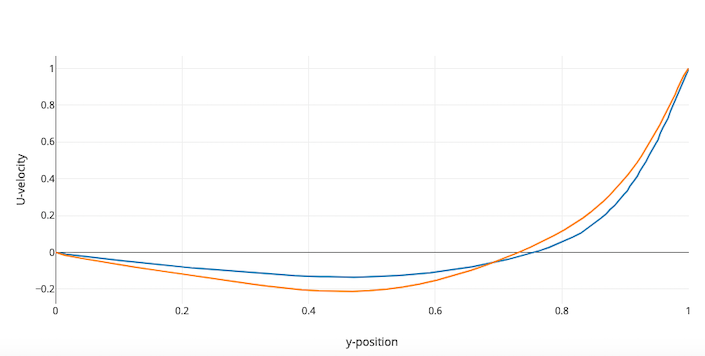comparison

The curves are not exactly the same because the barbatruc solver is light weighted.

The blue curve is from the simulation and the orange curve is from the figure 5 (with AR=1) of the CFD SIMULATIONS OF LID DRIVEN CAVITY FLOW AT MODERATE REYNOLDS NUMBER, by Reyad Omari, Department of Mathematics, Al-Balqa Applied University, European Scientific Journal May 2013 edition vol.9, No.15 ISSN: 1857 – 7881 (Print) e - ISSN 1857- 7431 22

To have a better graph, one can try to:

• refine the mesh
• modify the pressure solver

Indeed, there are two main problems:

• the cavity has singularities
• there might be an issue of mass conservation, indeed the solver of Poisson did not converge enough and therefore mass conservation is not completely achieved